St. Ignace Public Library

#### Non-Fiction Study Guide- Mathematics

###### BooksBuilding Blocks of Mathematics- Addition by Joseph Midthun A graphic nonfiction volume that introduces critical basic addition conceptsBuilding Blocks of Mathematics- Division By Joseph Midthun A graphic nonfiction volume that introduces critical division conceptsBuilding Blocks of Mathematics- Fractions by Joseph Midthun A graphic nonfiction volume that introduces critical fraction concepts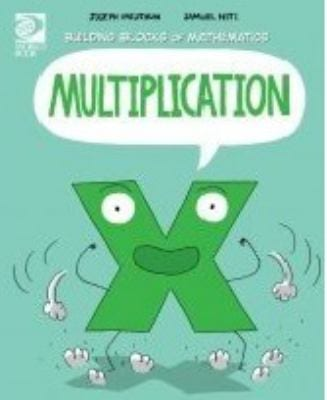Building Blocks of Mathematics- Multiplication by Joseph Midthun A graphic nonfiction volume that introduces critical multiplication concepts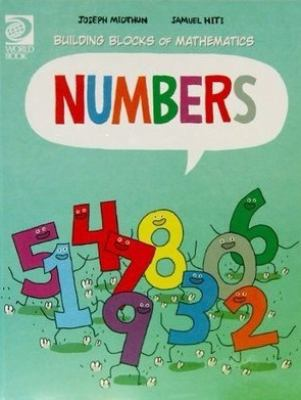Building Blocks of Mathematics- Number by Joseph Midthun A graphic nonfiction volume that introduces critical number conceptsBuilding Blocks of Mathematics- Subtraction by Joseph Midthun A graphic nonfiction volume that introduces critical subtraction concepts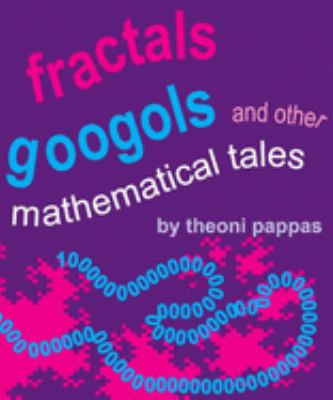Fractals, Googols and other Mathematical Tales by Theoni Pappas Includes short stories and discussions which present such mathematical concepts as decimals, tangrams, number lines, and fractals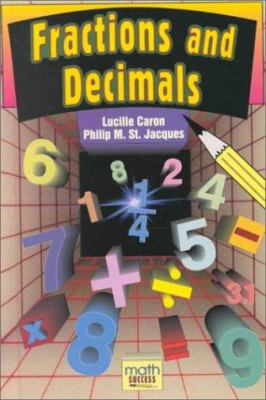Fractions and Decimals by Lucille Caron Explains how to add, subtract, multiply, and divide fractions and decimals; introduces estimating techniques; and includes many examplesPercents and Ratios by Lucille Caron Explains the meanings of percents and ratios, discusses calculation techniques, and includes many examples Introduces basic algebra, including integers and variables, explains problem solving techniques, and includes many examples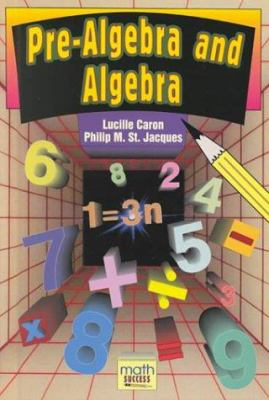Pre-Algebra and Algebra by Lucille Caron Introduces basic algebra, including integers and variables, explains problem solving techniques, and includes many examples
###### Practice Tests and Tutorials

6th – 8th Grade Math Tutorials

Expressions and Equations Practice Tests

Functions Practice Tests

Geometry Practice Tests

Number and Quantity Practice Tests

Ratios and Proportional Relationship Practice Tests

Statistics and Probability Practice Tests

• ### Hours

Monday: 10 AM – 5 PM
Tuesday: 10 AM – 5 PM
Wednesday: 10 AM – 7 PM
Thursday: 10 AM – 5 PM
Friday: 10 AM – 5 PM
Saturday: 10 AM – 3 PM

• ### Events & Activities

1. #### Friends of the Library Meeting

October 19 @ 5:15 pm - 6:00 pm
2. #### Drop in Arts & Crafts

October 20 @ 2:00 pm - 5:00 pm